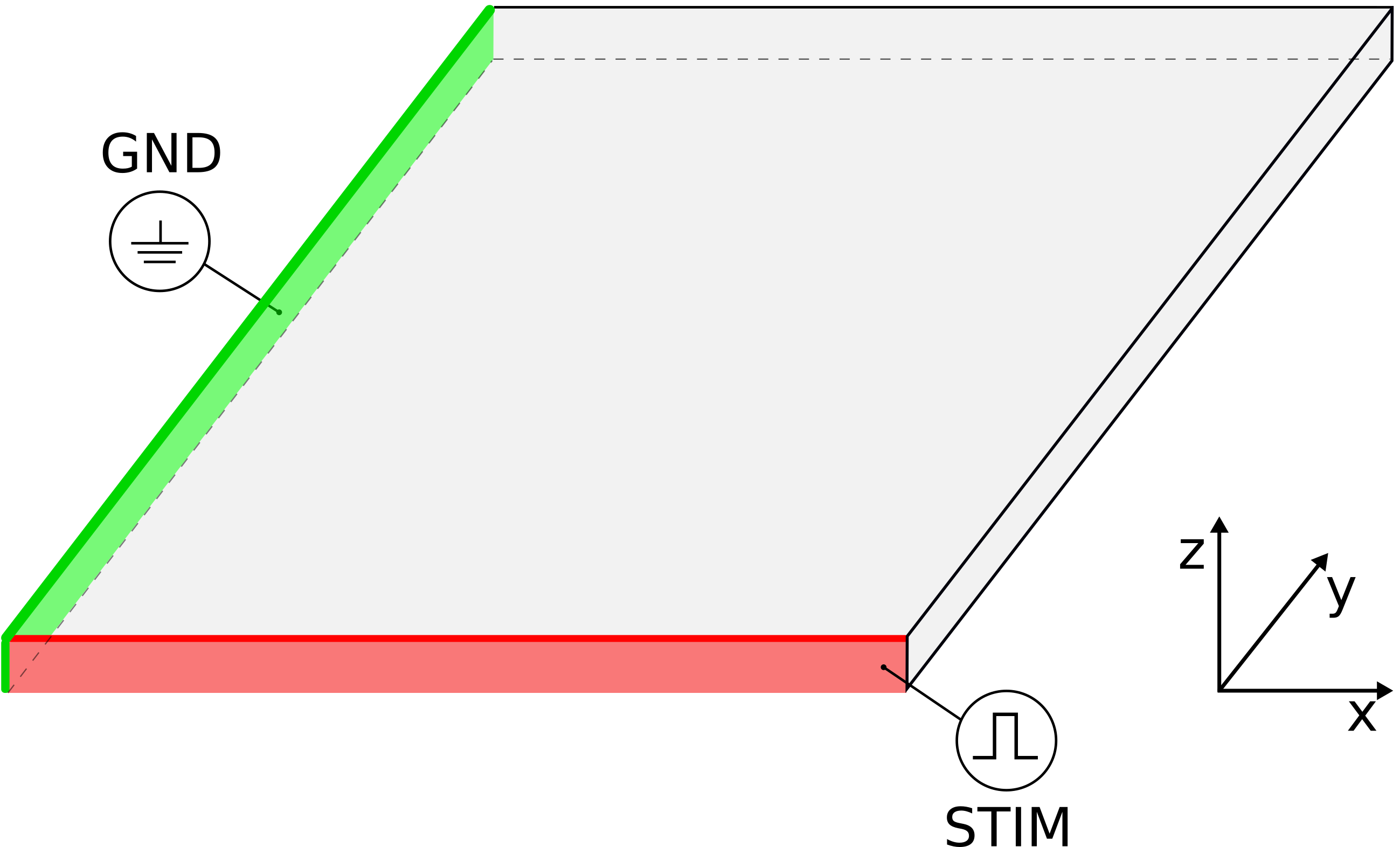# Laplace Solver

See code in GitLab.
Author: Anton J Prassl anton.prassl@medunigraz.at

## Introduction

Computing Laplace-Dirichlet maps provide an elegant tool for describing the distance between defined boundaries. Specialized software routines exploiting these maps in order to assign ventricular fibers and sheets or to determine the set of elements to receive heterogeneous conductivities are frequently used in the carputils framework. To run the experiments of this example change directories as follows:

cd \${TUTORIALS}/02_EP_tissue/13_laplace

## Experimental Setup

The geometry and the electrodes are defined as follows:An extracellular voltage stimulus (STIM) is applied to the lower boundary of a quadratic tetrahedral FE model with dimensions 10.0mm x 0.1mm x 10.0mm. The ground electrode (GND) is assigned to the left face side of mesh.

## Problem-specific openCARP Parameters

The relevant part of the .par file for this example is shown below:

experiment           = 2        # perform Laplace solve only
bidomain             = 1

# ground electrode
stimulus.x0       =   -50.   # par-file units are always microns!
stimulus.xd       =   100.
stimulus.y0       =   -50.
stimulus.yd       = 10100.
stimulus.z0       =   -50.
stimulus.zd       =   200.
stimulus.stimtype =     3    # extracellular ground

# stimulus electrode
stimulus.x0       =   -50.
stimulus.xd       = 10100.
stimulus.y0       =   -50.
stimulus.yd       =   100.
stimulus.z0       =   -50.
stimulus.zd       =   200.
stimulus.stimtype =     2    # extracellular voltage
stimulus.duration =     1.
stimulus.strength =     1.

# set isotropic conductivities everywhere
num_gregions         = 1
gregion.g_il      = 1
gregion.g_it      = 1
gregion.g_el      = 1
gregion.g_et      = 1

## Experiment

To run this experiment, do

./run.py --visualize

Note

• Set uniform conductivities everywhere to not disturb the Laplace solution
• Exclude any geometry parts (e.g. bath) you do not need the Laplace solution for. meshtool<mesh-data-extraction> will help you to reintegrate any computed features back into its 'parent'.
• openCARP needs the bidomain flag to be set to 1. Otherwise the program will abort with a hint to change this specific setting.
• One may scale the values of the Laplace solution through setting the stimulus strengths to e.g. 0 (stimulus, GND) and 1 (stimulus.strength) as shown above.
• The example collects stimulation nodes by defining an inclosing volume (x0,x0+xd,y0,y0+yd,z0,z0+zd). Alternatively, vertices can directly be addressed by including a vertex file<vertex-file>.

Expected result:

## Nodal Boundary Conditions

Instead of having to define a different stimulus for each different Dirichlet value, specific nodes can be assigned different values in one file. The relevant parameters to change are below:

stimulus.stimtype =     2    # extracellular voltage
stimulus.vtx_file =     BCs
stimulus.vtx_fcn  =     1
stimulus.strength =     1.

When stimulus.vtx_fcn is non-zero, the vtx_file, BCs.vtx in this case, has vertex adjustment format in which the nodal strength is specified along with the node number. The nodal strengths in the file are then multiplied by stimulus.strength.

© Copyright 2020 openCARP project    Supported by DFG and EuroHPC    Contact    Imprint and data protection